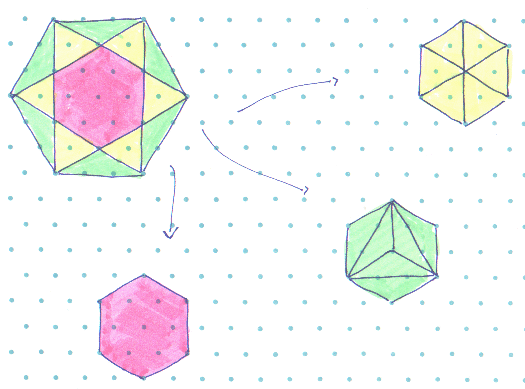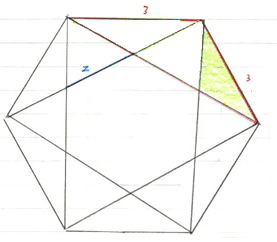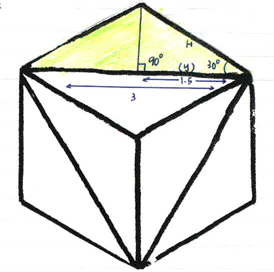#### You may also like### Tennis

A tennis ball is served from directly above the baseline (assume the ball travels in a straight line). What is the minimum height that the ball can be hit at to ensure it lands in the service area?### How Big?

If the sides of the triangle in the diagram are 3, 4 and 5, what is the area of the shaded square?### Triangular Tantaliser

Draw all the possible distinct triangles on a 4 x 4 dotty grid. Convince me that you have all possible triangles.

# Hex

##### Age 11 to 14 Challenge Level:

Malcolm Findlay from Madras College in St Andrews, Scotland has solved the first part of this problem:Izumi Tomioka, Carol Chow and Priscilla Luk from The Mount School in York solved the second part of the problem:

The original hexagon has sides of length 3 units and we need to work out $x$, the lengths of the sides of the smaller hexagons.The hexagon below has been made from 6 of the shaded triangles above.
We need to find the length of one of the sides of this hexagon.Splitting the shaded triangle into half gives us a right angled triangle such that: $$\cos30 = {1.5\over H}$$ $$H = {1.5\over \cos 30}$$ $$H = 1.73$$

Andrei Lazanu (aged 12) from School 205 in Bucharest, Romania, solved both parts of the problem.

This is how he tackled the second part:

To calculate the lengths of the sides of the smaller hexagons I used the following notations:

l for the length of side of the great hexagon
a for the length of side of the small hexagon

I used the following notation:

Triangle ACE is equilateral, because its sides are congruent.
So, angle EAC is $60$°.

Angle FAB is $120$°, since each angle of a regular hexagon is $120$°.

Triangle AEF is congruent with triangle ACB, having all sides congruent. They are also isosceles triangles.
This means that each of the angles FAE and CAB is $30$°.

Therefore angle EAB is $90$°.

Triangle AMN is also equilateral, because it has a $60$° angle (MAN) and AM = AN.
Triangle ANB is isosceles, so AN and NB are congruent.

Therefore, in the right angled triangle MAB,
MA = MN = NB = $a$

AB has length $l$

Applying the Pythagorean Theorem: $$l^2 = (a + a)^2 - a^2$$ $$l^2 = 4a^2 - a^2$$ $$l^2 = 3a^2$$ $$l = a \sqrt{3}$$

If the length of the side of the great hexagon is 3 units long $l = 3$

Therefore $a = \sqrt{3}$ units

An alternative way of calculating "a" takes into account the first part of the problem:
the area of the great hexagon is three times the area of the small one.

For a hexagon of side $l$, the area is calculated as 6 times the area of an equilateral triangle of side $l$,
this means that the area of the great hexagon is:

 $6{l^2 \sqrt3\over 4} = {l^2 3\sqrt3\over2}$ $(1)$

The 3 smaller hexagons of side "a" have a total area of:

 $3{a^2 3\sqrt3\over 2} = {a^2 9\sqrt3\over2}$ $(2)$

Since, $(1)$ and $(2)$ represent the same area, $3a^2 = l$

which is the same as we found above.